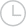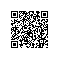# 斐波那契数列-问答-阿里云开发者社区-阿里云

## 斐波那契数列珍宝珠2019-11-19 15:12:12342

``````F0 = 0     (n=0)
F1 = 1    (n=1)
Fn = F[n-1]+ F[n-2](n=>2)
``````取消 提交回答

• 珍宝珠
2019-11-19 15:14:03

程序源代码：

``````方法一
#!/usr/bin/python
# -*- coding: UTF-8 -*-

def fib(n):
a,b = 1,1
for i in range(n-1):
a,b = b,a+b
return a

# 输出了第10个斐波那契数列
print fib(10)

``````
``````方法二
#!/usr/bin/python
# -*- coding: UTF-8 -*-

# 使用递归
def fib(n):
if n==1 or n==2:
return 1
return fib(n-1)+fib(n-2)

# 输出了第10个斐波那契数列
print fib(10)

``````

以上实例输出了第10个斐波那契数列，结果为：

``````55

``````
``````方法三
如果你需要输出指定个数的斐波那契数列，可以使用以下代码：

#!/usr/bin/python
# -*- coding: UTF-8 -*-

def fib(n):
if n == 1:
return 
if n == 2:
return [1, 1]
fibs = [1, 1]
for i in range(2, n):
fibs.append(fibs[-1] + fibs[-2])
return fibs

# 输出前 10 个斐波那契数列
print fib(10)

``````

以上程序运行输出结果为：

``````[1, 1, 2, 3, 5, 8, 13, 21, 34, 55]
``````
0 0使用钉钉扫一扫加入圈子
+ 订阅